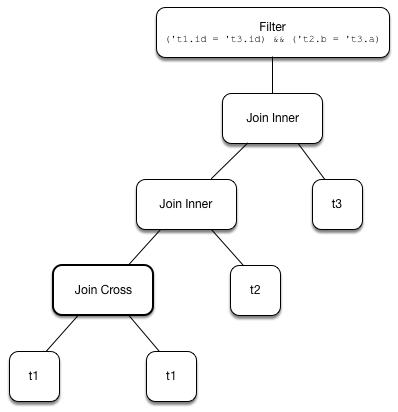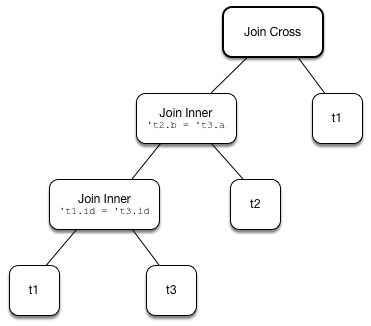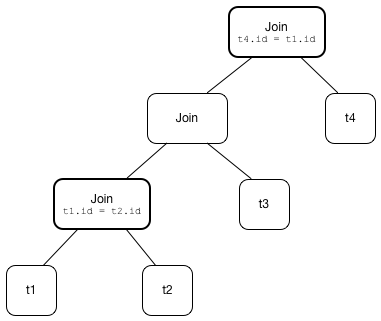## ReorderJoin Logical Optimization — Reordering Inner and Cross Joins

`ReorderJoin` is a logical optimization for join reordering.

`ReorderJoin` applies the join optimizations on a logical plan with 2 or more inner and cross joins with at least one join condition.

 Caution FIXME A diagram of a logical plan tree before and after the rule.

Technically, `ReorderJoin` is a Catalyst rule for transforming logical plans, i.e. `Rule[LogicalPlan]`.

``````// Build analyzed logical plan with at least 3 joins and zero or more filters
val large = spark.range(2 * belowBroadcastJoinThreshold)
val tiny = Seq(1,2,3,4,5).toDF("id")

crossJoin(large). // <-- CROSS JOIN of two fairly big datasets
join(tiny).
val plan = q.queryExecution.analyzed
scala> println(plan.numberedTreeString)
00 Filter (id#0L = cast(id#9 as bigint))
01 +- Join Inner
02    :- Join Cross
03    :  :- Range (0, 10485759, step=1, splits=Some(8))
04    :  +- Range (0, 20971518, step=1, splits=Some(8))
05    +- Project [value#7 AS id#9]
06       +- LocalRelation [value#7]

// Apply ReorderJoin rule
// ReorderJoin alone is (usually?) not enough
// Let's go pro and create a custom RuleExecutor (i.e. a Optimizer)
import org.apache.spark.sql.catalyst.rules.RuleExecutor
import org.apache.spark.sql.catalyst.plans.logical.LogicalPlan
import org.apache.spark.sql.catalyst.analysis.EliminateSubqueryAliases
object Optimize extends RuleExecutor[LogicalPlan] {
import org.apache.spark.sql.catalyst.optimizer._
val batches =
Batch("EliminateSubqueryAliases", Once, EliminateSubqueryAliases) ::
Batch("Operator Optimization", FixedPoint(maxIterations = 100),
ConvertToLocalRelation,
PushDownPredicate,
PushPredicateThroughJoin) :: Nil
}
val preOptimizedPlan = Optimize.execute(plan)
// Note Join Cross as a child of Join Inner
scala> println(preOptimizedPlan.numberedTreeString)
00 Join Inner, (id#0L = cast(id#9 as bigint))
01 :- Join Cross
02 :  :- Range (0, 10485759, step=1, splits=Some(8))
03 :  +- Range (0, 20971518, step=1, splits=Some(8))
04 +- LocalRelation [id#9]

// Time...for...ReorderJoin!
import org.apache.spark.sql.catalyst.optimizer.ReorderJoin
val optimizedPlan = ReorderJoin(preOptimizedPlan)
scala> println(optimizedPlan.numberedTreeString)
00 Join Cross
01 :- Join Inner, (id#0L = cast(id#9 as bigint))
02 :  :- Range (0, 10485759, step=1, splits=Some(8))
03 :  +- LocalRelation [id#9]
04 +- Range (0, 20971518, step=1, splits=Some(8))

// ReorderJoin works differently when the following holds:
// * cboEnabled is disabled
import org.apache.spark.sql.internal.SQLConf.STARSCHEMA_DETECTION
spark.sessionState.conf.setConf(STARSCHEMA_DETECTION, true)

spark.sessionState.conf.cboEnabled``````

`ReorderJoin` is part of the Operator Optimizations fixed-point batch in the standard batches of the Catalyst Optimizer.

### Applying ReorderJoin Rule To Logical Plan (Executing ReorderJoin) — `apply` Method

``apply(plan: LogicalPlan): LogicalPlan``
 Note `apply` is part of Rule Contract to apply a rule to a logical plan.

`apply` traverses the input logical plan down and finds the following logical operators for flattenJoin:

 Note `apply` uses `ExtractFiltersAndInnerJoins` Scala extractor object (using unapply method) to "destructure" a logical plan to its logical operators.

### Creating Join Logical Operator (Possibly as Child of Filter Operator) — `createOrderedJoin` Method

``createOrderedJoin(input: Seq[(LogicalPlan, InnerLike)], conditions: Seq[Expression]): LogicalPlan``

`createOrderedJoin` takes a collection of pairs of a logical plan and the join type with join condition expressions and…​FIXME

 Note `createOrderedJoin` makes sure that the `input` has at least two pairs in the `input`.
 Note `createOrderedJoin` is used recursively when `ReorderJoin` is applied to a logical plan.

#### "Two Logical Plans" Case

For two joins exactly (i.e. the `input` has two logical plans and their join types), `createOrderedJoin` partitions (aka splits) the input condition expressions to the ones that can be evaluated within a join and not.

`createOrderedJoin` determines the join type of the result join. It chooses inner if the left and right join types are both inner and cross otherwise.

`createOrderedJoin` creates a Join logical operator with the input join conditions combined together using `And` expression and the join type (inner or cross).

If there are condition expressions that could not be evaluated within a join, `createOrderedJoin` creates a Filter logical operator with the join conditions combined together using `And` expression and the result join operator as the child operator.

``````import org.apache.spark.sql.catalyst.expressions.Expression
import org.apache.spark.sql.catalyst.expressions.Literal
val a: Expression = Literal("a")
val b: Expression = Literal("b")
// Use Catalyst DSL to compose expressions
import org.apache.spark.sql.catalyst.dsl.expressions._
val cond1 = a === b

// RowNumber is Unevaluable so it cannot be evaluated within a join
import org.apache.spark.sql.catalyst.expressions.RowNumber
val rn = RowNumber()
import org.apache.spark.sql.catalyst.expressions.Unevaluable
assert(rn.isInstanceOf[Unevaluable])
val cond2 = rn === Literal(2)

val cond3 = Literal.TrueLiteral

// Use Catalyst DSL to create logical plans
import org.apache.spark.sql.catalyst.dsl.plans._
val t1 = table("t1")
val t2 = table("t2")

// Use input with exactly 2 pairs
import org.apache.spark.sql.catalyst.plans.logical.LogicalPlan
import org.apache.spark.sql.catalyst.plans.{Cross, Inner, InnerLike}
val input: Seq[(LogicalPlan, InnerLike)] = (t1, Inner) :: (t2, Cross) :: Nil
val conditions: Seq[Expression] = cond1 :: cond2 :: cond3 :: Nil

import org.apache.spark.sql.catalyst.optimizer.ReorderJoin
val plan = ReorderJoin.createOrderedJoin(input, conditions)
scala> println(plan.numberedTreeString)
00 'Filter (row_number() = 2)
01 +- 'Join Cross, ((a = b) && true)
02    :- 'UnresolvedRelation `t1`
03    +- 'UnresolvedRelation `t2```````

#### "Three Or More Logical Plans" Case

For three or more logical plans in the `input`, `createOrderedJoin` takes the first plan and tries to find another that has at least one matching join condition, i.e. a logical plan with the following:

1. Output attributes together with the first plan’s output attributes are the superset of the references of a join condition expression (i.e. both plans are required to resolve join references)

2. References of the join condition cannot be evaluated using the first plan’s or the current plan’s output attributes (i.e. neither the first plan nor the current plan themselves are enough to resolve join references)Figure 1. createOrderedJoin with Three Joins (Before)Figure 2. createOrderedJoin with Three Joins (After)
``````// HACK: Disable symbolToColumn implicit conversion
// It is imported automatically in spark-shell (and makes demos impossible)
// implicit def symbolToColumn(s: Symbol): org.apache.spark.sql.ColumnName
implicit def symbolToColumn(ack: ThatWasABadIdea) = ack

import org.apache.spark.sql.catalyst.plans.logical.LocalRelation
import org.apache.spark.sql.catalyst.dsl.expressions._
import org.apache.spark.sql.catalyst.dsl.plans._
// Note analyze at the end to analyze the queries
val p1 = LocalRelation('id.long, 'a.long, 'b.string).as("t1").where("id".attr =!= 0).select('id).analyze
val p2 = LocalRelation('id.long, 'b.long).as("t2").analyze
val p3 = LocalRelation('id.long, 'a.string).where("id".attr > 0).select('id, 'id * 2 as "a").as("t3").analyze

// The following input and conditions are equivalent to the following query
val _p1 = Seq((0,1,"one")).toDF("id", "a", "b").as("t1").where(col("id") =!= 0).select("id")
val _p2 = Seq((0,1)).toDF("id", "b").as("t2")
val _p3 = Seq((0,"one")).toDF("id", "a").where(col("id") > 0).select(col("id"), col("id") * 2 as "a").as("t3")
val _plan = _p1.
as("p1").
crossJoin(_p1).
join(_p2).
join(_p3).
where((col("p1.id") === col("t3.id")) && (col("t2.b") === col("t3.a"))).
queryExecution.
analyzed
import org.apache.spark.sql.catalyst.planning.ExtractFiltersAndInnerJoins
val Some((plans, conds)) = ExtractFiltersAndInnerJoins.unapply(_plan)

import org.apache.spark.sql.catalyst.plans.logical.LogicalPlan
import org.apache.spark.sql.catalyst.plans.{Cross, Inner, InnerLike}
val input: Seq[(LogicalPlan, InnerLike)] = Seq(
(p1, Cross),
(p1, Cross),
(p2, Inner),
(p3, Inner))

// (left ++ right).outputSet > expr.references
// ! expr.references > left.outputSet
// ! expr.references > right.outputSet
val c1 = p1_id === p3_id
val c2 = p2_b === p3_a

// A condition has no references or the references are not a subset of left or right plans
// A couple of assertions that createOrderedJoin does internally
assert(c1.references.nonEmpty)
assert(!c1.references.subsetOf(p1.outputSet))
assert(!c1.references.subsetOf(p3.outputSet))
val refs = p1.analyze.outputSet ++ p3.outputSet
assert(c1.references.subsetOf(refs))

import org.apache.spark.sql.catalyst.expressions.Expression
val conditions: Seq[Expression] = Seq(c1, c2)

assert(input.size > 2)
assert(conditions.nonEmpty)

import org.apache.spark.sql.catalyst.optimizer.ReorderJoin
val plan = ReorderJoin.createOrderedJoin(input, conditions)
scala> println(plan.numberedTreeString)
00 'Join Cross
01 :- Join Inner, (b#553L = a#556L)
02 :  :- Join Inner, (id#549L = id#554L)
03 :  :  :- Project [id#549L]
04 :  :  :  +- Filter NOT (id#549L = cast(0 as bigint))
05 :  :  :     +- LocalRelation <empty>, [id#549L, a#550L, b#551]
06 :  :  +- Project [id#554L, (id#554L * cast(2 as bigint)) AS a#556L]
07 :  :     +- Filter (id#554L > cast(0 as bigint))
08 :  :        +- LocalRelation <empty>, [id#554L, a#555]
09 :  +- LocalRelation <empty>, [id#552L, b#553L]
10 +- Project [id#549L]
11    +- Filter NOT (id#549L = cast(0 as bigint))
12       +- LocalRelation <empty>, [id#549L, a#550L, b#551]``````

`createOrderedJoin` takes the plan that has at least one matching join condition if found or the next plan from the `input` plans.

`createOrderedJoin` partitions (aka splits) the input condition expressions to expressions that meet the following requirements (aka join conditions) or not (aka others):

1. Expression references being a subset of the output attributes of the left and the right operators

2. Can be evaluated within a join

`createOrderedJoin` creates a Join logical operator with:

1. Left logical operator as the first operator from the `input`

2. Right logical operator as the right as chosen above

3. Join type as the right’s join type as chosen above

4. Join conditions combined together using `And` expression

`createOrderedJoin` calls itself recursively with the following:

1. `input` logical joins as a new pair of the new `Join` and `Inner` join type with the remaining logical plans (all but the right)

2. `conditions` expressions as the others conditions (all but the join conditions used for the new join)Figure 3. createOrderedJoin with Three Joins
``````import org.apache.spark.sql.catalyst.expressions.Expression
import org.apache.spark.sql.catalyst.expressions.AttributeReference
import org.apache.spark.sql.types.LongType
val t1_id: Expression = AttributeReference(name = "id", LongType)(qualifier = Some("t1"))
val t2_id: Expression = AttributeReference(name = "id", LongType)(qualifier = Some("t2"))
val t4_id: Expression = AttributeReference(name = "id", LongType)(qualifier = Some("t4"))
// Use Catalyst DSL to compose expressions
import org.apache.spark.sql.catalyst.dsl.expressions._
val cond1 = t1_id === t2_id

// RowNumber is Unevaluable so it cannot be evaluated within a join
import org.apache.spark.sql.catalyst.expressions.RowNumber
val rn = RowNumber()
import org.apache.spark.sql.catalyst.expressions.Unevaluable
assert(rn.isInstanceOf[Unevaluable])
import org.apache.spark.sql.catalyst.expressions.Literal
val cond2 = rn === Literal(2)

// That would hardly appear in the condition list
// Just for the demo
val cond3 = Literal.TrueLiteral

val cond4 = t4_id === t1_id

// Use Catalyst DSL to create logical plans
import org.apache.spark.sql.catalyst.dsl.plans._
val t1 = table("t1")
val t2 = table("t2")
val t3 = table("t3")
val t4 = table("t4")

// Use input with 3 or more pairs
import org.apache.spark.sql.catalyst.plans.logical.LogicalPlan
import org.apache.spark.sql.catalyst.plans.{Cross, Inner, InnerLike}
val input: Seq[(LogicalPlan, InnerLike)] = Seq(
(t1, Inner),
(t2, Inner),
(t3, Cross),
(t4, Inner))
val conditions: Seq[Expression] = cond1 :: cond2 :: cond3 :: cond4 :: Nil

import org.apache.spark.sql.catalyst.optimizer.ReorderJoin
val plan = ReorderJoin.createOrderedJoin(input, conditions)
scala> println(plan.numberedTreeString)
00 'Filter (row_number() = 2)
01 +- 'Join Inner, ((id#11L = id#12L) && (id#13L = id#11L))
02    :- 'Join Cross
03    :  :- 'Join Inner, true
04    :  :  :- 'UnresolvedRelation `t1`
05    :  :  +- 'UnresolvedRelation `t2`
06    :  +- 'UnresolvedRelation `t3`
07    +- 'UnresolvedRelation `t4```````

### Extracting Filter and Join Operators from Logical Plan — `unapply` Method (of ExtractFiltersAndInnerJoins)

``unapply(plan: LogicalPlan): Option[(Seq[(LogicalPlan, InnerLike)], Seq[Expression])]``

`unapply` extracts Filter (with an inner or cross join) or Join logical operators (per the input logical plan) to…​FIXME

 Note `unapply` is a feature of the Scala programming language to define extractor objects that take an object and try to give the arguments back. This is most often used in pattern matching and partial functions.
1. For a Filter logical operator with a cross or inner Join child operator, `unapply` flattenJoin on the `Filter`.

2. For a Join logical operator, `unapply` flattenJoin on the `Join`.

``````val d1 = Seq((0, "a"), (1, "b")).toDF("id", "c")
val d2 = Seq((0, "c"), (2, "b")).toDF("id", "c")
val q = d1.join(d2, "id").where(\$"id" > 0)
val plan = q.queryExecution.analyzed

scala> println(plan.numberedTreeString)
00 Filter (id#34 > 0)
01 +- Project [id#34, c#35, c#44]
02    +- Join Inner, (id#34 = id#43)
03       :- Project [_1#31 AS id#34, _2#32 AS c#35]
04       :  +- LocalRelation [_1#31, _2#32]
05       +- Project [_1#40 AS id#43, _2#41 AS c#44]
06          +- LocalRelation [_1#40, _2#41]

// Let's use Catalyst DSL instead so the plan is cleaner (e.g. no Project in-between)
// We could have used logical rules to clean up the plan
// Leaving the cleaning up as a home exercise for you :)
import org.apache.spark.sql.catalyst.dsl.plans._
val t1 = table("t1")
val t2 = table("t2")
import org.apache.spark.sql.catalyst.expressions.Expression
import org.apache.spark.sql.catalyst.expressions.Literal
val id: Expression = Literal("id")
import org.apache.spark.sql.catalyst.dsl.expressions._
import org.apache.spark.sql.catalyst.plans.Cross
val plan = t1.join(t1, joinType = Cross).join(t2).where(id > 0)
scala> println(plan.numberedTreeString)
00 'Filter (id > 0)
01 +- 'Join Inner
02    :- 'Join Cross
03    :  :- 'UnresolvedRelation `t1`
04    :  +- 'UnresolvedRelation `t1`
05    +- 'UnresolvedRelation `t2`

import org.apache.spark.sql.catalyst.planning.ExtractFiltersAndInnerJoins
// Option[(Seq[(LogicalPlan, InnerLike)], Seq[Expression])]
val Some((plans, conditions)) = ExtractFiltersAndInnerJoins.unapply(plan)

assert(plans.size > 2)
assert(conditions.nonEmpty)

CAUTION: FIXME``````
 Note `unapply` is used exclusively when `ReorderJoin` is executed, i.e. applied to a logical plan.

### Flattening Consecutive Joins — `flattenJoin` Method (of ExtractFiltersAndInnerJoins)

``````flattenJoin(plan: LogicalPlan, parentJoinType: InnerLike = Inner):
(Seq[(LogicalPlan, InnerLike)], Seq[Expression])``````

`flattenJoin` branches off per the input logical `plan`:

• For an inner or cross Join logical operator, `flattenJoin` calls itself recursively with the left-side of the join and the type of the join, and gives:

1. The logical plans from recursive `flattenJoin` with the right-side of the join and the right join’s type

2. The join conditions from `flattenJoin` with the conditions of the join

• For a Filter with an inner or cross Join child operator, `flattenJoin` calls itself recursively on the join (that simply removes the `Filter` "layer" and assumes an inner join) and gives:

1. The logical plans from recursive `flattenJoin`

2. The join conditions from `flattenJoin` with `Filter`'s conditions

• For all other logical operators, `flattenJoin` gives the input `plan`, the current join type (an inner or cross join) and the empty join condition.

In either case, `flattenJoin` splits conjunctive predicates, i.e. removes `And` expressions and gives their child expressions.

``````// Use Catalyst DSL to create a logical plan
// Example 1: One cross join
import org.apache.spark.sql.catalyst.dsl.plans._
val t1 = table("t1")
import org.apache.spark.sql.catalyst.dsl.expressions._
val id = "id".expr
import org.apache.spark.sql.catalyst.plans.Cross
val plan = t1.join(t1, joinType = Cross)
scala> println(plan.numberedTreeString)
00 'Join Cross
01 :- 'UnresolvedRelation `t1`
02 +- 'UnresolvedRelation `t1`

import org.apache.spark.sql.catalyst.planning.ExtractFiltersAndInnerJoins
val (plans, conditions) = ExtractFiltersAndInnerJoins.flattenJoin(plan)
assert(plans.size == 2)
assert(conditions.size == 0)

// Example 2: One inner join with a filter
val t2 = table("t2")
val plan = t1.join(t2).where("t1".expr === "t2".expr)
scala> println(plan.numberedTreeString)
00 'Filter (t1 = t2)
01 +- 'Join Inner
02    :- 'UnresolvedRelation `t1`
03    +- 'UnresolvedRelation `t2`

val (plans, conditions) = ExtractFiltersAndInnerJoins.flattenJoin(plan)
assert(plans.size == 2)
assert(conditions.size == 1)

// Example 3: One inner and one cross join with a compound filter
val plan = t1.
join(t1, joinType = Cross).
join(t2).
where("t2.id".expr === "t1.id".expr && "t1.id".expr > 10)
scala> println(plan.numberedTreeString)
00 'Filter ((t2.id = t1.id) && (t1.id > 10))
01 +- 'Join Inner
02    :- 'Join Cross
03    :  :- 'UnresolvedRelation `t1`
04    :  +- 'UnresolvedRelation `t1`
05    +- 'UnresolvedRelation `t2`

val (plans, conditions) = ExtractFiltersAndInnerJoins.flattenJoin(plan)
assert(plans.size == 3)
assert(conditions.size == 2)

// Example 4
val t3 = table("t3")
val plan = t1.
join(t1, joinType = Cross).
join(t2).
where("t2.id".expr === "t1.id".expr && "t1.id".expr > 10).
join(t3.select(star())).  // <-- just for more fun
where("t3.id".expr === "t1.id".expr)
scala> println(plan.numberedTreeString)
00 'Filter (t3.id = t1.id)
01 +- 'Join Inner
02    :- 'Filter ((t2.id = t1.id) && (t1.id > 10))
03    :  +- 'Join Inner
04    :     :- 'Join Cross
05    :     :  :- 'UnresolvedRelation `t1`
06    :     :  +- 'UnresolvedRelation `t1`
07    :     +- 'UnresolvedRelation `t2`
08    +- 'Project [*]
09       +- 'UnresolvedRelation `t3`

val (plans, conditions) = ExtractFiltersAndInnerJoins.flattenJoin(plan)
assert(plans.size == 4)
assert(conditions.size == 3)

// Example 5: Join under project is no longer consecutive
val plan = t1.
join(t1, joinType = Cross).
select(star()). // <-- separates the cross join from the other joins
join(t2).
where("t2.id".expr === "t1.id".expr && "t1.id".expr > 10).
join(t3.select(star())).
where("t3.id".expr === "t1.id".expr)
scala> println(plan.numberedTreeString)
00 'Filter (t3.id = t1.id)
01 +- 'Join Inner
02    :- 'Filter ((t2.id = t1.id) && (t1.id > 10))
03    :  +- 'Join Inner
04    :     :- 'Project [*]
05    :     :  +- 'Join Cross
06    :     :     :- 'UnresolvedRelation `t1`
07    :     :     +- 'UnresolvedRelation `t1`
08    :     +- 'UnresolvedRelation `t2`
09    +- 'Project [*]
10       +- 'UnresolvedRelation `t3`

val (plans, conditions) = ExtractFiltersAndInnerJoins.flattenJoin(plan)
assert(plans.size == 3) // <-- one join less due to Project
assert(conditions.size == 3)

// Example 6: Join on right-hand side is not considered
val plan = t1.
join(
t1.join(t2).where("t2.id".expr === "t1.id".expr && "t1.id".expr > 10), // <-- join on RHS
joinType = Cross).
join(t2).
where("t2.id".expr === "t1.id".expr && "t1.id".expr > 10)
scala> println(plan.numberedTreeString)
00 'Filter ((t2.id = t1.id) && (t1.id > 10))
01 +- 'Join Inner
02    :- 'Join Cross
03    :  :- 'UnresolvedRelation `t1`
04    :  +- 'Filter ((t2.id = t1.id) && (t1.id > 10))
05    :     +- 'Join Inner
06    :        :- 'UnresolvedRelation `t1`
07    :        +- 'UnresolvedRelation `t2`
08    +- 'UnresolvedRelation `t2`

val (plans, conditions) = ExtractFiltersAndInnerJoins.flattenJoin(plan)
assert(plans.size == 3) // <-- one join less due to being on right side
assert(conditions.size == 2)``````
 Note `flattenJoin` is used recursively when `ReorderJoin` is destructures a logical plan (when executed).